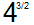## Simplification of numbers with Fractional Exponents

The exponent (or Power, or Index) of a number says how many times to use a number in a multiplication.
For Example- 2² = 2 × 2,
Or,        5³ = 5 × 5 × 5

### FractionalExponents-

But what if the exponent is in Fraction?
fractional exponent is an alternate notation for expressing powers and roots together. For example, the following are equivalent. We write the power in the numerator and the index of the root in the denominator.
*The fractional exponent of a number is actually its Root. like Square root, Cube root, 4th root, 5th root, etc. see-

### Prove-

Let's see an example proving that-
Recall- remember What the laws of exponent tell us how to handle exponent when we multiply-
see this example- x² x²  = (xx) (xx) = xxxx = x

it shows that-
Now, Try this on a fractional exponent-
Example:-  what is 1/2 power of 5 × 1/2 power of  5?

and, The number which gives another number when multiplied by itself, Called? The Square Root!

### What about More complicated Fractions?

so we just learned about the power of 1/2, which is quite simple Right! but what about the more complicated fractional exponents like-Actually, This is saying to do a Cube, and a Square root of 4, in any order. (Remember! in any order)
Let me explain this to you-